Goseeko blog# What are Feedback Amplifiers ?

There are basically two categories in which the Feedback Amplifiers are divided

Positive Feedback Amplifiers– The feedback amplifiers in which the source and feedback signals are in the same phase are called positive feedback amplifiers. The strength of the input signal is increased because of such feedback.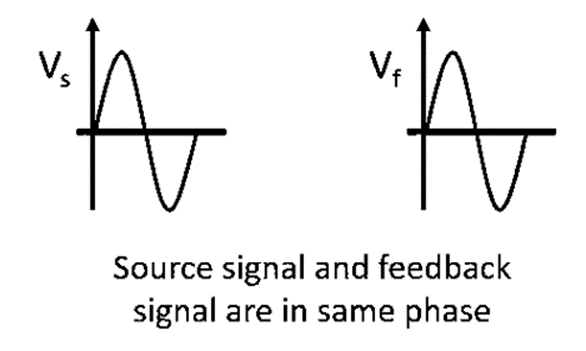Negative Feedback Amplifier– The negative feedback amplifiers are one in which the the source and feedback signals are out of phase. The strength of the input signal decreases because of such feedback.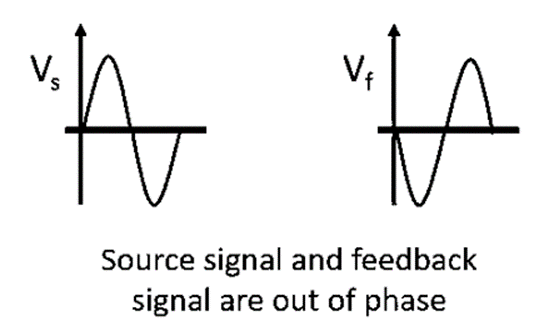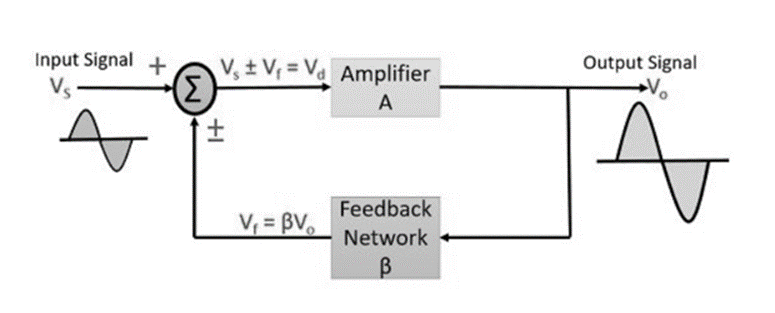As we see from the above figure the input voltage is represented by Vs with gain A, that produces an amplified signal, Vo.

Some part of this amplified signal is fed again as feedback which has a gain of β.  Vf, this signal is provided as the feedback to the summer or a mixer that resultantly produces either sum or difference of two signals depending on their phase relationship..

The gain of an amplifier is the ratio of output voltage or current to the input voltage or current. The basic types of amplifiers on the basis of feedback are below.

## Types on basis of feedback

Voltage Series Feedback: In this type of arrangement the feedback which is applied to the input is connected in shunt. The portion of output which is connected to the input voltage is in series with the input voltage. Hence, we can say that the feedback is in shunt by means of output and in series by means of the input.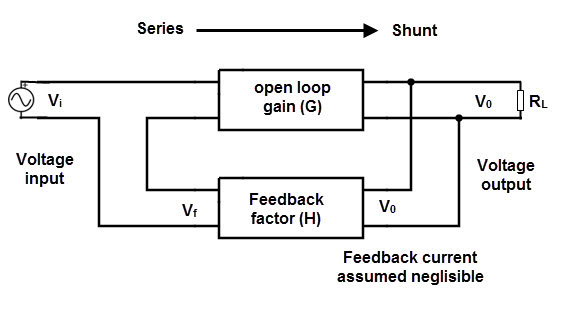Voltage shunt Feedback: In this type of arrangement the feedback which is applied to the input is connected in series. The portion of output which is connected to the input voltage is in shunt with the input voltage. Hence, we can conclude that the feedback is said to be connected in series by means of output and in shunt by means of the input.Current Series Feedback:  The current series feedback circuit is one which has the feedback circuit which is in shunt for output and in series to the input signal. As the connection is in series with the input the value of impedance is high. The value of impedance is high as the output being in series.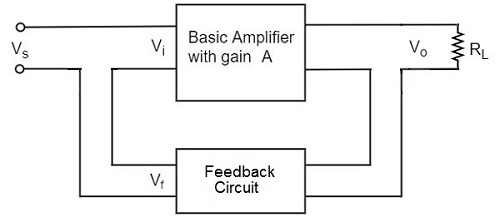Current Shunt Feedback:

The current shunt feedback circuit is one which has the feedback circuit and also which is in series for output and in shunt to the input signal. Then the connection is in parallel with the input the value of impedance is low. Also, the output being in series results in a high value of impedance.

Due to the parallel connection with the input the value of impedance is low at this point. Whereas the output connected in terms of series makes the value of impedance high.

## Comparison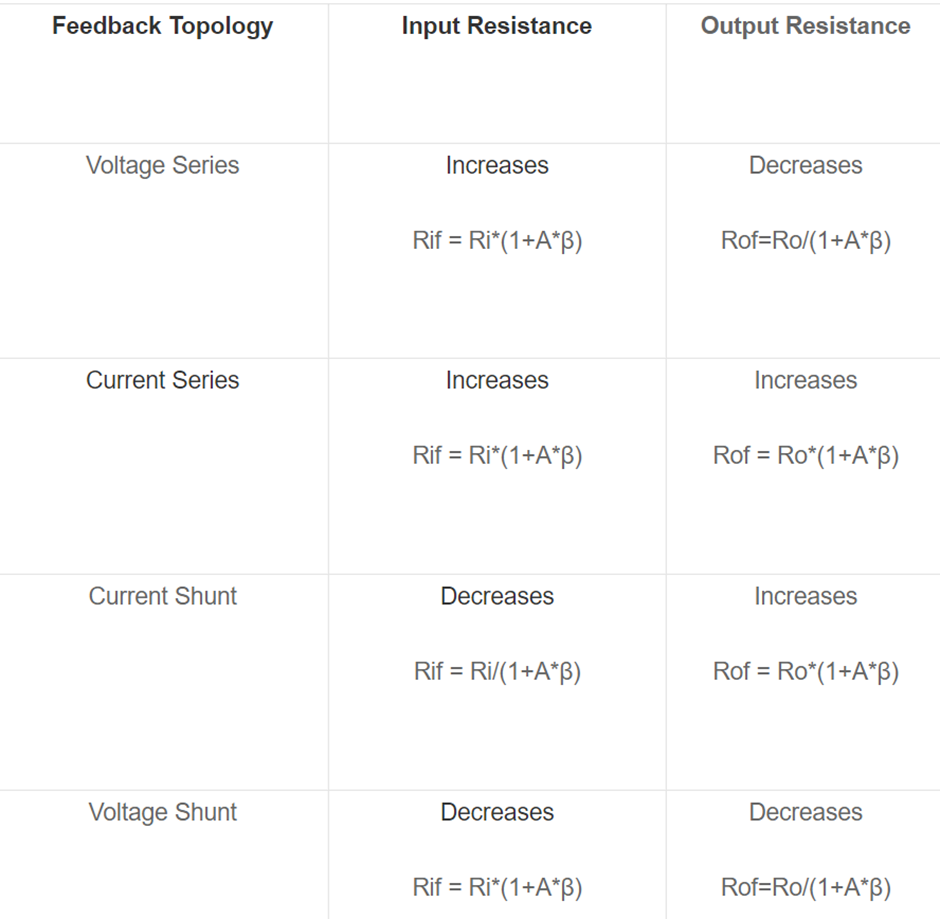Interested in learning about similar topics? Here are a few hand-picked blogs for you!